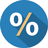# PercentageQuantitative Aptitude Questions and Answers for Banking Examinations on Percentage with explanation. Each question is followed by a video solution for better understanding and practice

# If 20% of A = 50% of B, Then what per cent of A is B ?

If 20% of A = 50% of B, Then what per cent of A is B ? [A]15% [B]25% [C]30% [D]40% Show Answer 40% 20% of A = 50% of B Let B is x% of A. Hence option [D] is the correct answer.

# 30% of x is 72. The value of x is :

30% of x is 72. The value of x is : [A]240 [B]216 [C]480 [D]640 Show Answer 240 30% of x is 72 Hence option [A] is the correct answer.

# Half of 1 per cent, written as a decimal, is :

Half of 1 per cent, written as a decimal, is : [A]0.2 [B]0.005 [C]0.02 [D]0.05 Show Answer 0.005 Hence option [B] is the correct answer.

# 65g is what per cent of 2 kg?

65g is what per cent of 2 kg? [A] [B] [C] [D] Show Answer Required Percentage = Hence option [C] is the correct answer.

# 0.01 is what per cent of 0.1?

0.01 is what per cent of 0.1? [A] [B] [C]100 [D]10 Show Answer 10 Let Hence option [D] is the correct answer.